Describing motion along a line

This free course is available to start right now. Review the full course description and key learning outcomes and create an account and enrol if you want a free statement of participation.

Free course

5.2 The equations of uniformly accelerated motion

Equations 22, 23 and 24 provide a complete description of uniformly accelerated motion. By combining them appropriately, it is possible to solve a wide class of problems concerning the kinematics of uniformly accelerated motion. Nonetheless, those particular equations are not always the best starting point for the most common problems. For example, it is often the case that we want to know the displacement from the initial position after some specified period of constant acceleration, rather than the final position x. In such circumstances it is useful to subtract x﻿0 from both sides of Equation 22 and use the definition s﻿x﻿ = xx﻿0 to write the resulting equation as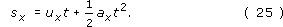More significantly, it is often the case that we need to find the final velocity v﻿x when all we are given is the (constant) acceleration ax, the initial velocity ux and the displacement sx. The problem can be solved using Equations 23 and 25, but doing so involves finding the duration of the motion t, which is not required as part of the answer. It would be more convenient to use an equation that related v﻿x to a﻿x, u﻿x and s﻿x directly, thus avoiding the need to work out t altogether. Fortunately, it is possible to find such an equation by using a standard mathematical procedure called elimination.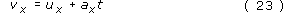The first step in the process is to identify a set of equations that contain the variables we want to relate, along with at least one variable we can eliminate. In this case we want to eliminate t from Equations 23 and 25. The second step usually involves rearranging one of the equations so that the unwanted variable is isolated on the left-hand side, thus becoming the subject of that equation. In this case, we can subtract u﻿x from both sides of Equation 23, divide both sides by a﻿x and then interchange the two sides to give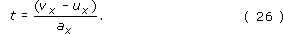Having obtained this relation from one of the equations, the third step is to use it to eliminate the unwanted variable from all the other equations. In our case this means replacing t by (﻿v﻿﻿﻿xu﻿x﻿)﻿/﻿a﻿x throughout Equation 25, so we obtain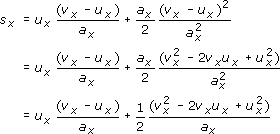so after a little algebra, we obtain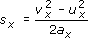which can be rearranged to give the result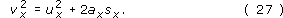Equations 23, 25 and 27 are the most frequently used equations of uniformly accelerated motion and are usually referred to collectively as the constant acceleration equations (or the uniform acceleration equations).

Constant (or uniform) acceleration equations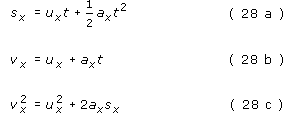It follows from these equations that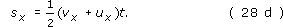Remember, these are not universal equations that describe every form of motion. They apply only to situations in which the acceleration a﻿x is constant.

Question 20

Starting from the constant acceleration equations, use the elimination procedure to derive Equation 28d.

In this case we wish to eliminate ax from Equations 28a and 28b. One way is to rearrange Equation 28b (subtracting ux from both sides and dividing both sides by t) to obtain: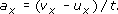Substituting this into Equation 28a gives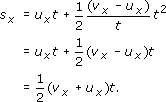Question 21

Give a graphical interpretation of Equation 28d in terms of the area under a velocity-time graph for the case of uniformly accelerated motion.

Graphically, sx is the signed area under the velocity-time graph between the given times. In this case those times are t = 0 when the initial velocity is ux and some later time t when the velocity is vx. Since the velocity-time graph for uniformly accelerated motion is a straight line of fixed gradient, the area required will always be either a trapezium (above or below the axis) or a pair of triangles. (Figure 37 shows a particular case.)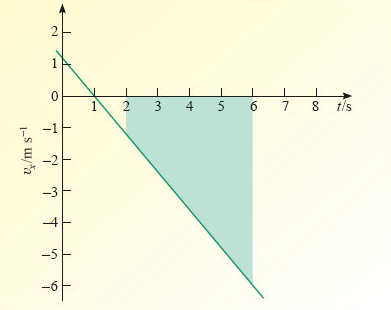Figure 37: The velocity-time graph for Self-assessment question 19

Question 22

An object has a final velocity of 30.0 m s﻿−﻿1, after accelerating uniformly at 2.00 m s﻿−﻿2 over a displacement of 20.0 m. (a) What was the initial speed of the object? (b) For how long was the object accelerated?

(a) In this case we know sx = 20 m, vx = 30 m s−1 and ax = 2 m s−2, and we need to find ux. As a first step we should make ux the subject of an equation that involves the known quantities. Rearranging Equation 28c gives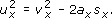Taking the square root of each sideSubstituting the given values,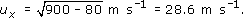(b) The duration of the acceleration is given by Equation 26 as t = (vxux)/ax, which was itself obtained by rearranging constant acceleration equations. Substituting the relevant values gives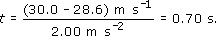Question 23

A train accelerates uniformly along a straight track at 2.00 m s﻿−﻿2 from an initial velocity of 4.00 m s﻿−﻿1 to a final velocity of 16.00 m s﻿−﻿1. What is the train's displacement from its initial position at the end of this interval?

Using Equation 28c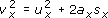with ux = 4.00 m s−1, vx = 16.0 m s−1 and ax = 2.00 m s−2, we obtain, after rearranging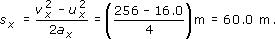S207_2